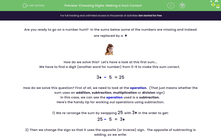# Choosing Digits: Making a Sum Correct

In this worksheet, students choose the missing digits needed to make the given statements correct.Key stage:  KS 2

Curriculum topic:   Number: Addition and Subtraction

Curriculum subtopic:   Solve Add/Subtract Problems to 1000

Difficulty level:#### Worksheet Overview

Are you ready to go on a number hunt?  In the problems below, some of the numbers are missing and have been replaced by aHow do we solve this?  Let's have a look at this first problem.

We have to find a digit (another word for number) from 0-9 to make this calculation correct:

3♦  -  5  = 25

How do we solve this question? First of all, we need to look at the operation.  (That just means whether the sum uses an addition, subtraction, multiplication or division sign)

In this case, we can see that the operation used is a subtraction

Here's the handy tip for working out operations using subtraction.

1) We re-arrange the calculation by swapping 25 with 3♦  to get:

25 -  5  =  3♦

2) Then we change the sign so that it uses the opposite (or inverse) sign.  The opposite of subtracting is adding, so we write:

25 + 5  =  3♦

3) Now we solve the sum:

25 + 5  =  30

so we now know that in this problem, the  ♦ meant 0

4) The last thing we do is put our number back into the original calculation to check our answer:

30  -  5  = 25

Now we move on to try to solve a problem where two numbers are missing.  Looks much harder?  Don't panic we can apply the same clever tip!

Let's look at the calculation below:

8  -  4  = 23

First of all, let's have a quick think about what each number represents?

8: In this number the 8 means 8 tens, and the ♦ stands for the ones.

4: In this number the  stands for the tens, and the stands for the 4 ones.

23:  In this number the 2 stands for 2 tens, and the 3 stands for three ones.

We need to start with one missing number first, so we'll start with the missing red number.  Ignore the green missing number for now!

The red missing number represents the ones.  So, for now, we're going to gather together all of the ones in the calculation above (the second number).

- 4 = 3

Do you remember what we did last time with a calculation where the operation was a subtraction?  We rearranged it first by swapping the first number and the number after the equals:

3 - 4 =

Then we replaced the subtraction sign with an addition sign (as this was the opposite):

3 + 4 =

Then we solved the sum:

3 + 4 = 7

Then we put it back in the original calculation to check it made sense:

- 4 = 3

We can put the 7 into our calculation below as we know this is our missing one...but remember...we have two missing numbers this time!

So now we have:

87  4  = 23

To find out our second missing number (the missing tens digit) we can do exactly the same process:

Gather the tens together:

8 -  = 2

Rearrange the sum by swapping the first number and the number after the equals sign to get:

-  = 8

Change the subtraction sign to an addition sign (as these are opposite) to give:

+  = 8

Solve the sum:

+ 6 = 8

Put this number back in the original calculation:

- 6 = 2

When we put the number back in our original calculation with two missing numbers we now have the statement 87  -  64  = 23

That's a lot to follow, but it really does get easier the more you practise.

Shall we have a go?

### What is EdPlace?

We're your National Curriculum aligned online education content provider helping each child succeed in English, maths and science from year 1 to GCSE. With an EdPlace account you’ll be able to track and measure progress, helping each child achieve their best. We build confidence and attainment by personalising each child’s learning at a level that suits them.

Get started